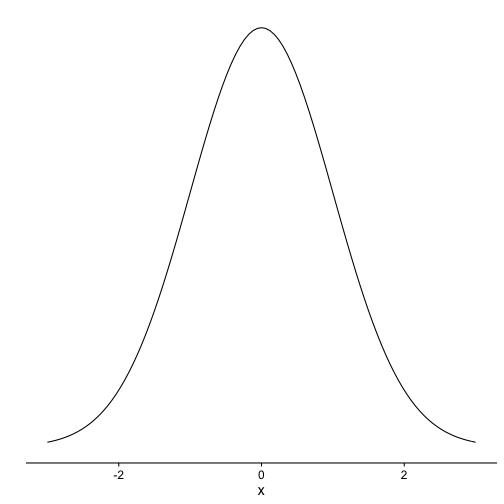# Simple way to plot a normal distribution with ggplot2

## August 30, 2016

Reading time ~1 minute

Plotting a normal distribution is something needed in a variety of situation: Explaining to students (or professors) the basic of statistics; convincing your clients that a t-Test is (not) the right approach to the problem, or pondering on the vicissitudes of life…

If you like ggplot2, you may have wondered what the easiest way is to plot a normal curve with ggplot2?

Here is one:

library(cowplot)

## Loading required package: ggplot2

##
## Attaching package: 'cowplot'

## The following object is masked from 'package:ggplot2':
##
##     ggsave

p1 <- ggplot(data = data.frame(x = c(-3, 3)), aes(x)) +
stat_function(fun = dnorm, n = 101, args = list(mean = 0, sd = 1)) + ylab("") +
scale_y_continuous(breaks = NULL)
p1Note that cowplot here is optional, and gives a more “clean” appearance to the plot. Without cowplot, ie., the standard theme of ggplot2, you will get (better restart your R session before running the next code):

library(ggplot2)

p1 <- ggplot(data = data.frame(x = c(-3, 3)), aes(x)) +
stat_function(fun = dnorm, n = 101, args = list(mean = 0, sd = 1)) + ylab("") +
scale_y_continuous(breaks = NULL)
p1### New bar stacking with ggplot 2.2.0

Recently, ggplot2 2.2.0 was released. Among other news, stacking bar plot was improved. Here is a short demonstration.Load libraries`...… Continue reading

#### Crashkurs zur Erstellung von Barplots für Umfrage-Daten

Published on November 13, 2016

#### Some thoughts (and simulation) on overfitting

Published on November 12, 2016